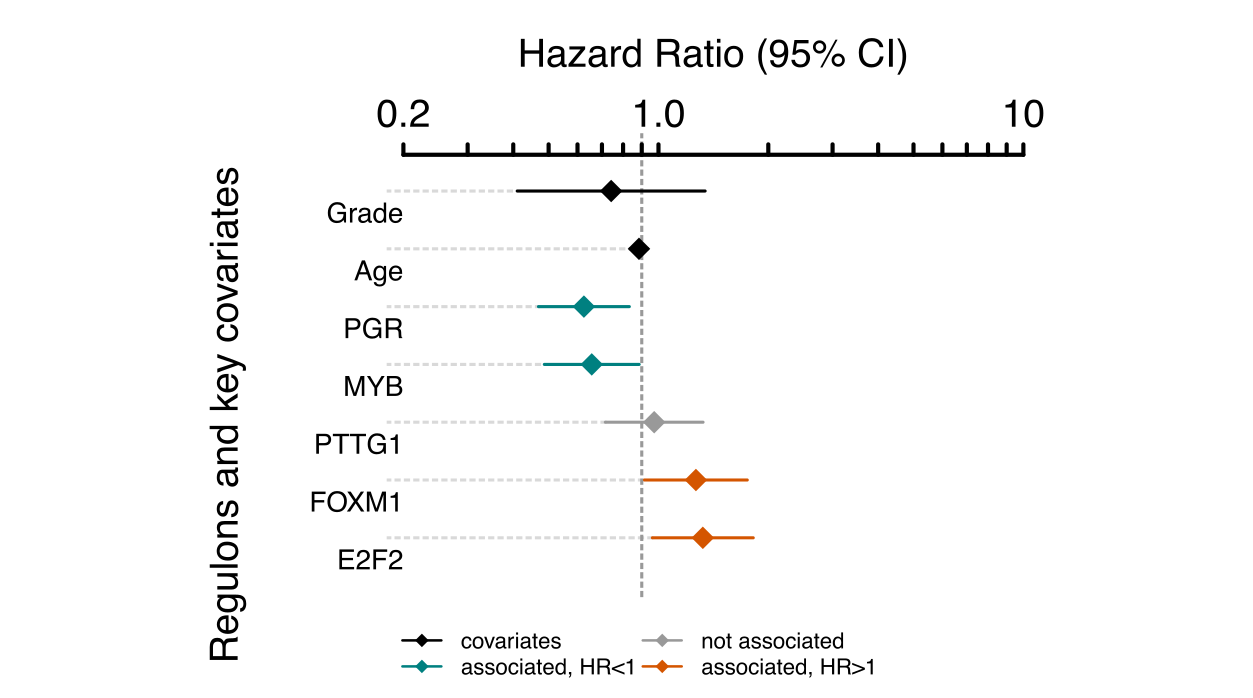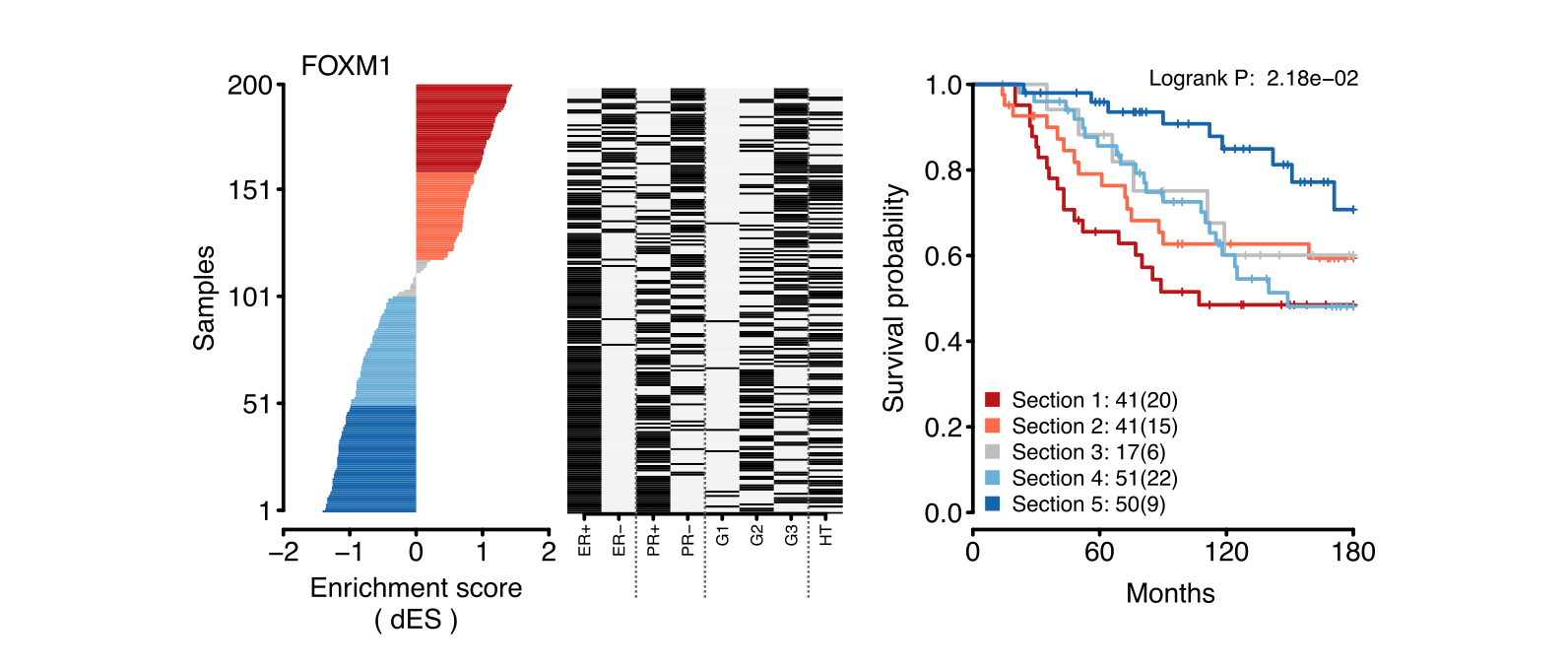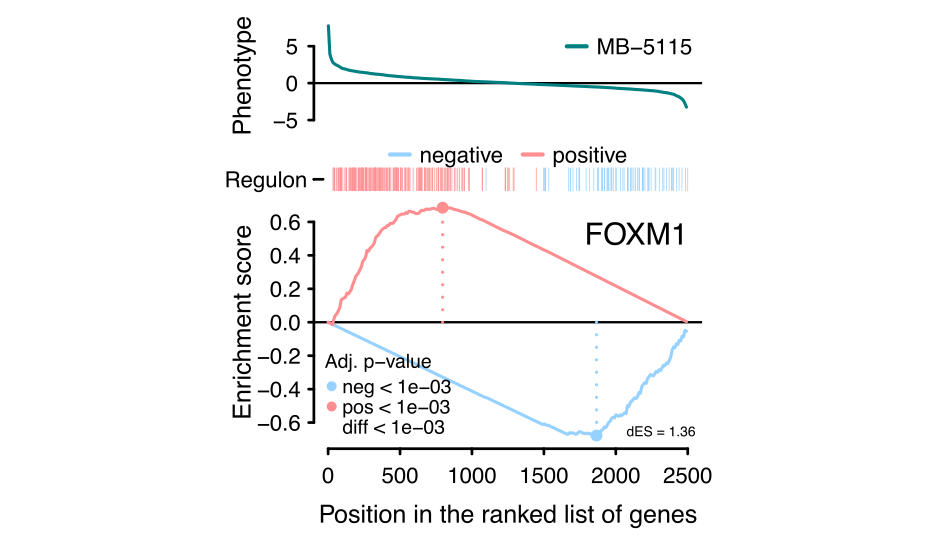# 1 Overview

Transcriptional networks are important tools to visualize complex biological systems that involve large groups of genes and multiple regulators. In a previous study, we have implemented the RTN R/Bioconductor package to reconstruct transcriptional regulatory networks (Fletcher et al. 2013). This package reconstructs regulons, consisting of a regulator and its target genes. A regulon can be further used to investigate, for example, the association of its expression on survival probabilities.

RTNsurvival is a tool for integrating regulons generated by the RTN package with survival information for the same set of samples used in the reconstruction of the transcriptional regulatory network. There are two main survival analysis pipelines: a Cox Proportional Hazards approach used to model regulons as predictors of survival time (Figure 1), and a Kaplan-Meier analysis showing the stratification of a cohort based on the regulon activity (Figure 2). For a given regulon, the 2-tailed GSEA approach is used to estimate regulon activity (differential Enrichment Score - dES) for each individual sample (Castro et al. 2016), and the dES distribution of all samples is then used to assess the survival statistics for the cohort. The plots can be fine-tuned to the user’s specifications.

# 2 Quick Start

## 2.1 Load regulons and survival data

This Vignette uses precomputed regulons available in the RTN package:

``````library(RTN)
data(stni, package="RTN")``````

The `stni` is a `TNI-class` object created from a subset of the expression data available in the `Fletcher2013b` package. The `stni` object contains a minimal toy reconstruction of 5 regulons (PGR, MYB, E2F2, FOXM1 and PTTG1).

More information about the parameters used to build the toy regulons can be viewed by calling the summary of the `stni` object.

``summary <- tni.get(stni, what = "summary")``

The RTNsurvival package provides a survival table for the samples in the `stni` object, including clinical data from the METABRIC study (Curtis et al. 2012) where the expression data was originally obtained.

``````library(RTNsurvival)
data(survival.data)``````

## 2.2 Preprocess input data

In order to run the analysis pipelines, the input data must be evaluated by the `tnsPreprocess` method in order to build a `TNS-class` object. Note that the survival table must be provided with time and event columns, and key covariates can also be specified for subsequent use in the Cox analysis.

``rtns <- tni2tnsPreprocess(stni, survivalData = survival.data, time = 1, event = 2, endpoint = 120, keycovar = c("Grade","Age"))``

## 2.3 Compute regulon activity

The survival analysis pipeline depends on the 2-tailed GSEA approach, which estimates regulon activity (dES) for all samples in the cohort. The `tnsGSEA2` function calls the `tni.gsea2` method available in the RTN package.

``rtns <- tnsGSEA2(rtns)``

## 2.4 Run the Cox analysis pipeline

Once the dES metric has been computed by `tnsGSEA2` function, then it is possible to run the Cox analysis.

The `tnsCox` method runs a Cox multivariate regression analysis and shows the proportional hazards of each of the specified regulons and the provided key covariates, indicating the contribution of each variable to survival (Figure 1). The method uses the Bioconductor survival package to fit the Cox model.

``````rtns <- tnsCox(rtns)
tnsPlotCox(rtns)``````Figure 1 - The plot shows the Hazard Ratio for all key covariates and regulons. Lines that are completely to the right of the grey line, shown in red, are positively associated with hazard. This means that samples with high expression of this regulon have poor prognosis. The further to the right or left of the grey line, the more significant is the association.

## 2.5 Run the Kaplan-Meier analysis pipeline

The `tnsKM` method generates a Kaplan-Meier plot, which consists of three panels put together: a ranked dES plot for the cohort, a status of key attributes plot (optional) and a Kaplan-Meier plot, showing survival curves for lower and higher dES samples (Figure 2).

``````rtns <- tnsKM(rtns)
tnsPlotKM(rtns, regs="FOXM1", attribs = list(c("ER+","ER-"),c("PR+","PR-"),c("G1","G2","G3"),"HT"))``````Figure 2 - The Kaplan-Meier plot for FOXM1 shows that samples with high regulon activity (red and pink) have poorer prognosis, as their survival probability is lower than the samples that have low regulon activity (light and dark blue).

# 3 Plot 2-tailed GSEA for individual samples

Individual sample differential enrichment analysis can be investigated using the `tnsPlotGSEA2` function. This will generate a 2-tailed GSEA plot for the differential expression of both positive and negative targets of a regulon (Figure 3). This step takes a little longer because the GSEA is recomputed for a selected regulon, and because `tnsPlotGSEA2` is a wrapper for the RTN function `tna.plot.gsea2`, which generates the GSEA plot.

``tnsPlotGSEA2(rtns, "MB-5115", regs = "FOXM1")``Figure 3 - The 2-tailed GSEA plot for the MB-5116 sample. It shows that the positive targets of the FOXM1 regulator are positively enriched, while the negative targets are negatively enriched.

# 4 Plot regulon activity for all samples

An overview of regulon activity can be obtained by plotting a heatmap with all evaluated samples and regulons. First, we need to obtain the matrix of dES values from the TNS object. Then, we can plot the heatmap using the `pheatmap` function from the pheatmap package. In this example, we also illustrate how to incorporate sample features from the survival data.

``````library(pheatmap)
enrichmentScores <- tnsGet(rtns, "regulonActivity")
survival.data <- tnsGet(rtns, "survivalData")
annotation_col <- survival.data[,c("ER+", "ER-")]
pheatmap(t(enrichmentScores\$dif),
annotation_col = annotation_col,
show_colnames = FALSE,
annotation_legend = FALSE)``````